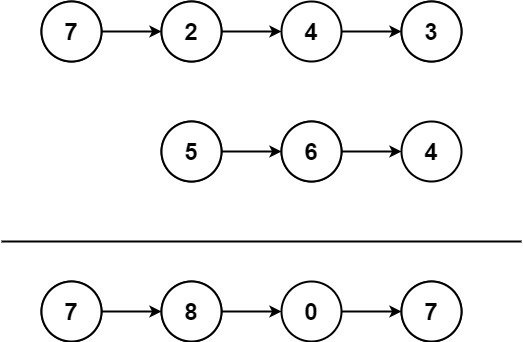# 445. Add Two Numbers II

Medium
You are given two non-empty linked lists representing two non-negative integers. The most significant digit comes first and each of their nodes contains a single digit. Add the two numbers and return the sum as a linked list.
You may assume the two numbers do not contain any leading zero, except the number 0 itself.
Example 1:Input: l1 = [7,2,4,3], l2 = [5,6,4]
Output:
[7,8,0,7]
Example 2:
Input: l1 = [2,4,3], l2 = [5,6,4]
Output:
[8,0,7]
Example 3:
Input: l1 = , l2 = 
Output:

Constraints:
• The number of nodes in each linked list is in the range `[1, 100]`.
• `0 <= Node.val <= 9`
• It is guaranteed that the list represents a number that does not have leading zeros.
Follow up: Could you solve it without reversing the input lists?

### 解題

Runtime: 10 ms, faster than 85.71%
Memory Usage: 5.5 MB, less than 19.5%
/**
* type ListNode struct {
* Val int
* Next *ListNode
* }
*/
func addTwoNumbers(l1 *ListNode, l2 *ListNode) *ListNode {
stack1 := make([]int, 0)
stack2 := make([]int, 0)
temp := l1
for temp != nil {
stack1 = append(stack1, temp.Val)
temp = temp.Next
}
temp = l2
for temp != nil {
stack2 = append(stack2, temp.Val)
temp = temp.Next
}
carry := 0
for len(stack1) != 0 || len(stack2) != 0 || carry > 0 {
sum := carry
if len(stack1) != 0 {
sum += stack1[len(stack1) - 1]
stack1 = stack1[:len(stack1) - 1]
}
if len(stack2) != 0 {
sum += stack2[len(stack2) - 1]
stack2 = stack2[:len(stack2) - 1]
}
carry = sum / 10
tmp := ListNode{ sum % 10, nil }